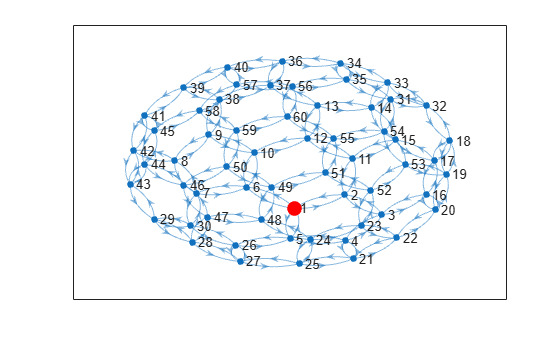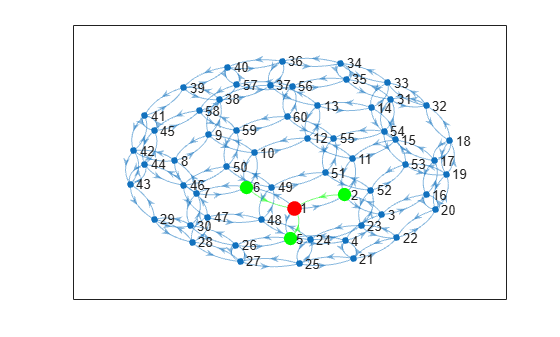# inedges

Incoming edges to node

## Syntax

``eid = inedges(G,nodeID)``
``[eid,nid] = inedges(G,nodeID)``

## Description

example

````eid = inedges(G,nodeID)` returns the indices of all incoming edges to node `nodeID` in directed graph `G`.```

example

````[eid,nid] = inedges(G,nodeID)` additionally returns the node IDs of the predecessor nodes connected to `nodeID` by the edges in `eid`.```

## Examples

collapse all

Create a multigraph with three nodes and four edges. Find the incoming edges of node 3.

```G = digraph([1 1 1 2],[2 2 3 3]); G.Edges```
```ans=4×1 table EndNodes ________ 1 2 1 2 1 3 2 3 ```
`eid = inedges(G,3)`
```eid = 2×1 3 4 ```
`G.Edges(eid,:)`
```ans=2×1 table EndNodes ________ 1 3 2 3 ```

Plot a graph and highlight the incoming edges and predecessors of a selected node.

Create and plot a directed graph using the `bucky` adjacency matrix. Highlight node 1 for reference.

```G = digraph(bucky); p = plot(G); highlight(p,1,'NodeColor','r','MarkerSize',10)```Determine the incoming edges and predecessors of node 1. Highlight these nodes and edges.

`[eid,nid] = inedges(G,1)`
```eid = 3×1 4 13 16 ```
```nid = 3×1 2 5 6 ```
`X = G.Edges(eid,:)`
```X=3×2 table EndNodes Weight ________ ______ 2 1 1 5 1 1 6 1 1 ```
```highlight(p,nid,'NodeColor','g','MarkerSize',9) highlight(p,'Edges',eid,'EdgeColor','g')```## Input Arguments

collapse all

Input graph, specified as a `digraph` object. Use `digraph` to create a directed graph object.

Example: `G = digraph([1 2],[2 3])`

Node identifier, specified as one of the values in this table.

ValueExample
Scalar node index`1`
Character vector node name`'A'`
String scalar node name`"A"`

Example: `inedges(G,1)`

Example: `inedges(G,'A')`

## Output Arguments

collapse all

Edge indices, returned as a column vector. You can use the edge indices to index into the edges table of the graph with `G.Edges(eid,:)`.

Node IDs of predecessors, returned as node indices if `nodeID` is numeric, or as node names if `nodeID` is a node name. Use `findnode(G,nid)` to convert node names into node indices. You can use node indices to index into the nodes table of the graph with `G.Nodes(nid,:)`.

The node IDs in `nid` are the same as those returned by the `predecessors` function. However, if there are multiple incoming edges from the same node, this node is listed more than once in `nid`.

## Tips

• By convention, for undirected graphs, all edges incident to a node are considered to be outgoing edges. Use `outedges` with undirected graphs.

• For graphs with multiple edges, `inedges` and `predecessors` can return arrays of different lengths, since there can be multiple incoming edges from some of the predecessors.

## Version History

Introduced in R2018a